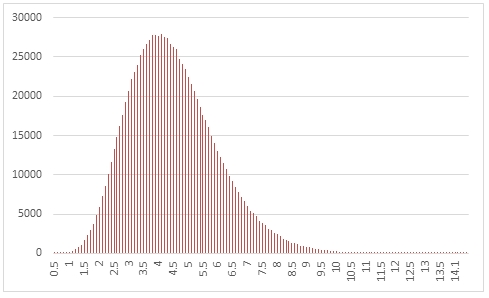# SQL Server radom gamma distribution

RANDGAMMA

Updated: 31 March 2014

Use the table-valued function RANDGAMMA to generate a sequence of random numbers from a gamma distribution with parameters @Shape and @Scale.
Syntax
SELECT * FROM [wctMath].[wct].[RANDGAMMA](
<@Rows, int,>
,<@Shape, float,>
,<@Scale, float,>)
Arguments
@Rows
the number of rows to generate. @Rows must be of the type int or of a type that implicitly converts to int.
@Shape
the shape parameter. @Shape must be of the type float or of a type that implicitly converts to float.
@Scale
the scale parameter. @Scale must be of the type float or of a type that implicitly converts to float.
Return Types
RETURNS TABLE (
[Seq] [int] NULL,
[X] [float] NULL
)
Remarks
·         @Shape must be greater than zero.
·         @Scale must be greater than zero.
·         If @Shape is NULL then @Shape is set to 1.
·         If @Scale is NULL then @Scale is set to 1.
·         If @Rows is less than 1 then no rows are returned.
Examples
In this example we create a sequence 1,000,000 random numbers rounded to one decimal place from a gamma distribution with @Shape = 9 and @Scale = 0, COUNT the results, paste them into Excel, and graph them.
SELECT
X,
COUNT(*) as [COUNT]
FROM (
SELECT
ROUND(X,1) as X
FROM
wct.RANDGAMMA(
1000000, --@Rows
9,       --@Shape
0.5      --@Scale
)
)n
GROUP BY
X
ORDER BY
X

This produces the following result.In this example we generate 1,000,000 random numbers from a gamma distribution with @Shape of 5 and @Scale of 2. We calculate the mean, standard deviation, skewness, and excess kurtosis from the resultant table and compare those values to the expected values for the distribution.
DECLARE @size as int = 1000000
DECLARE @Shape as float = 5
DECLARE @scale as float = 2
DECLARE @mean as float = @Shape*@Scale
DECLARE @var as float = @Shape*POWER(@Scale,2)
DECLARE @stdev as float = SQRT(@var)
DECLARE @skew as float = 2/SQRT(@Shape)
DECLARE @kurt as float = 6e+00/@Shape

SELECT
stat,
[RANDGAMMA],
[EXPECTED]
FROM (
SELECT
x.*
FROM (
SELECT
MIN(x) as min_GAMMA
,AVG(x) as mean_GAMMA
,MAX(x) as max_GAMMA
,STDEVP(x) as stdev_GAMMA
,wct.SKEWNESS_P(x) as skew_GAMMA
,wct.KURTOSIS_P(x) as kurt_GAMMA
FROM
wct.RANDGAMMA(@size,@Shape,@scale)
)n
CROSS APPLY(
VALUES
('RANDGAMMA','avg', mean_GAMMA),
('RANDGAMMA','stdev', stdev_GAMMA),
('RANDGAMMA','skew', skew_GAMMA),
('RANDGAMMA','kurt', kurt_GAMMA),
('EXPECTED','avg',@mean),
('EXPECTED','stdev',@stdev),
('EXPECTED','skew',@skew),
('EXPECTED','kurt',@kurt)
)x(fn_name,stat,val_stat)
)d
PIVOT(sum(val_stat) FOR fn_name in([RANDGAMMA],[EXPECTED])) P

This produces the following result (your result will be different).

 stat RANDGAMMA EXPECTED avg 9.998933155 10 kurt 1.21987376 1.2 skew 0.896956119 0.894427191 stdev 4.473389818 4.472135955

### SupportCopyright 2008-2023 Westclintech LLC         Privacy Policy        Terms of Service